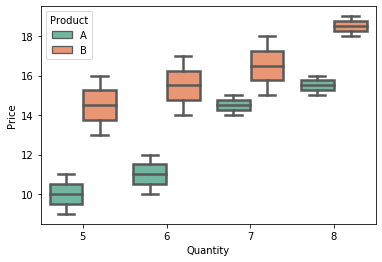# Seaborn 箱线图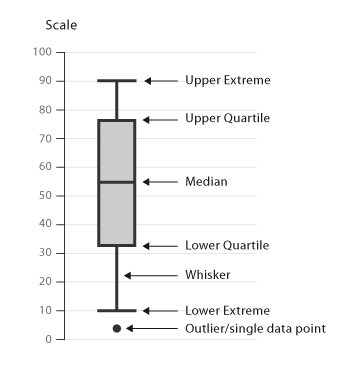`boxplot()` 函数可以有效地绘制数据集的分类值，也可以处理单个列表或数组向量。它与小提琴图非常相似。

``````import random
import numpy as np
import seaborn as sns
n = random.sample(range(0,50),30)
arr = np.array(n)
sns.boxplot(n)
``````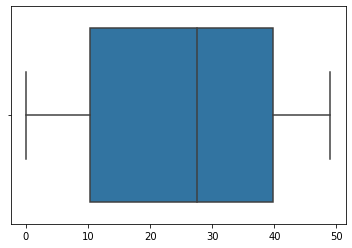``````import random
import numpy as np
import seaborn as sns
n = random.sample(range(0,50),30)
arr = np.array(n)
sns.boxplot(n)
sns.stripplot(n, color = 'red')
``````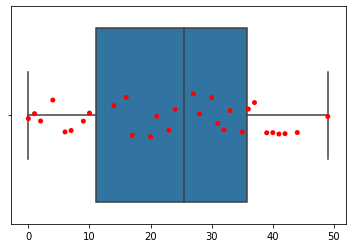``````import pandas as pd
import matplotlib.pyplot as plt
import seaborn as sns
df = pd.DataFrame({"Quantity": [5,6,7,8,5,6,7,8,5,6,7,8,5,6,7,8],
"Price": [9,10,15,16,13,14,15,18,11,12,14,15,16,17,18,19],
"Day" : [1,1,1,1,1,1,1,1,2,2,2,2,2,2,2,2],
"Product": ['A','A','A','A','B','B','B','B',
'A','A','A','A','B','B','B','B']})
sns.boxplot(data = df, y = "Price", x = "Quantity")
``````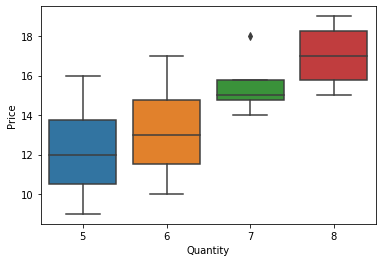``````import pandas as pd
import matplotlib.pyplot as plt
import seaborn as sns
df = pd.DataFrame({"Quantity": [5,6,7,8,5,6,7,8,5,6,7,8,5,6,7,8],
"Price": [9,10,15,16,13,14,15,18,11,12,14,15,16,17,18,19],
"Day" : [1,1,1,1,1,1,1,1,2,2,2,2,2,2,2,2],
"Product": ['A','A','A','A','B','B','B','B',
'A','A','A','A','B','B','B','B']})
sns.boxplot(data = df, y = "Price", x = "Quantity", hue = 'Product', linewidth = 2.5 , palette = 'Set2')
``````